# EdgeLock™ SE050: Plug & Trust Secure Element Family – Enhanced IoT security with maximum flexibility

## EdgeLock™ SE050: Securing tomorrow's IoT## EdgeLock SE050 Plug & Trust for IoT in HX2QFN20 package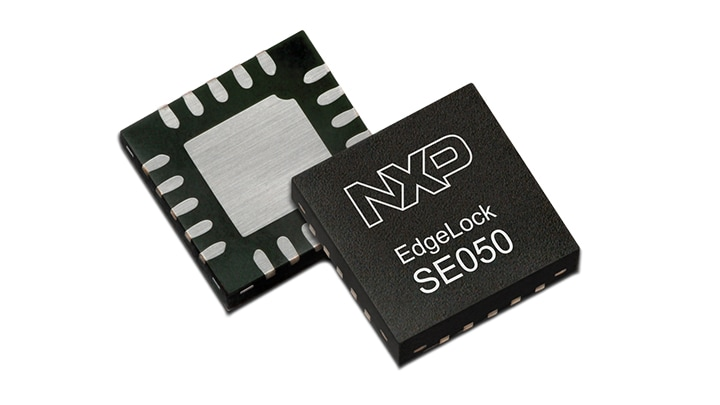## EdgeLock™ SE050 – Plug & Trust for Secure Sensor Authentication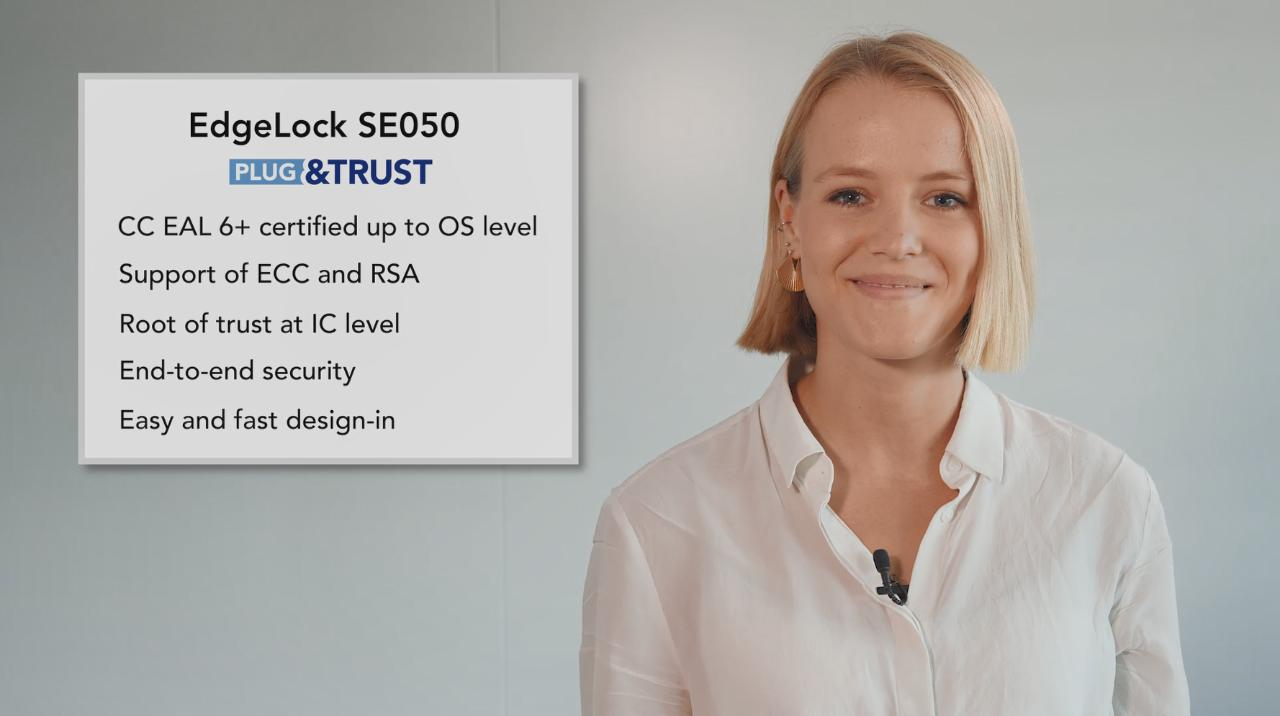## ‘Plug & Trust’ Security at Embedded World 2019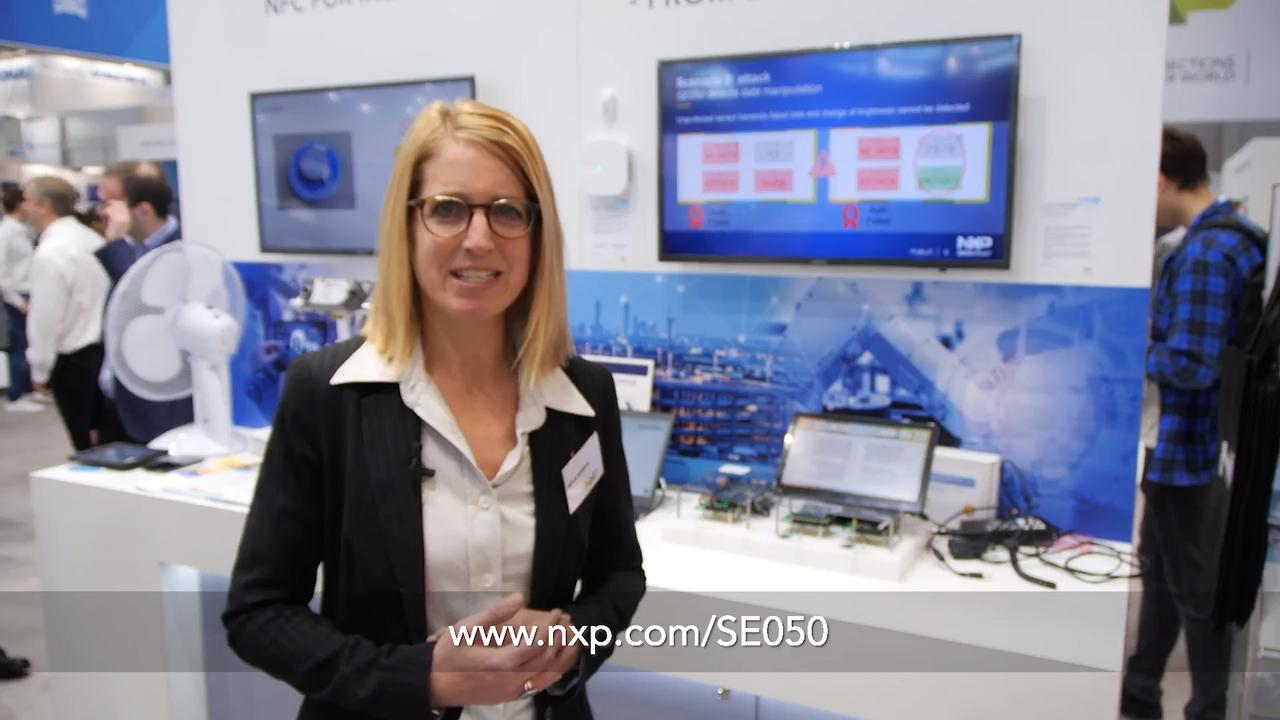## Secure Access Solutions Showcased at Embedded World 2019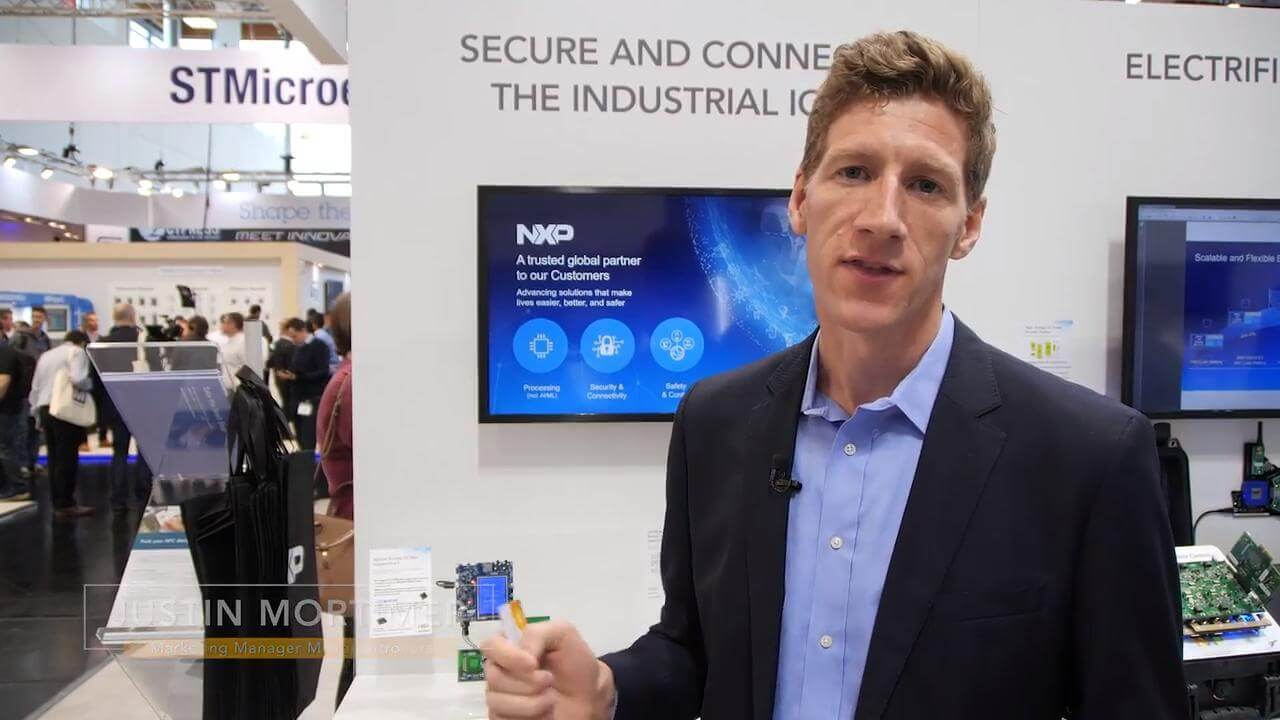## Demonstration EdgeLock™ SE050 Secure Element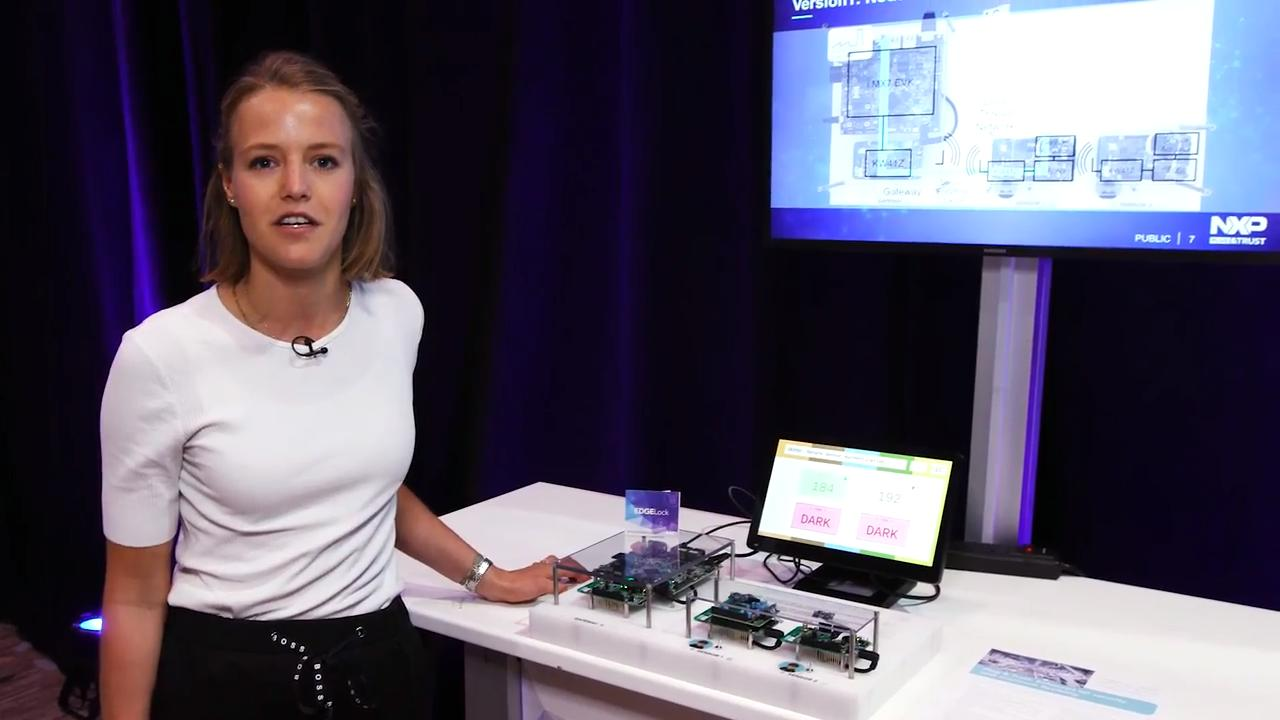.

## Comparison Table

SE050C1
SE050C2
SE050B1
SE050B2
SE050A1
SE050A2
OM-SE050ARD Dev Kit
RSA RSA (up to 4096) x x x x x
Supported Elliptic Curves NIST (192 to 521 bit) x x x x x
Brainpool (160 to 512 bit) x x x x x
Koblitz (160 to 256 bit) x x x x x
Twisted Edwards (for Ed25519) x x x
Montgomery (Curve25519) x x x
ECC Crypto Schemes ECDSA x x x x x
ECDH x x x x x
ECDHE x x x x x
ECDAA x x x
EdDSA x x x
Symmetric Crypto Algorithm 3DES (2K, 3K) x x x x x x x
AES (128, 192, 256) x x x x x x x
Hash Function SHA-1, SHA-224, SHA-256, SHA-384, SHA-512 x x x x x x x
MAC HMAC, CMAC x x x x x x x
Key Derivation (KDF) TLS KDF, TLS PSK x x x x x x x
MIFARE DESFire KDF x x x
Wi-Fi KDF (PBKDF2) x x x x x x x
OPC_UA KDF x x x x x x x
TPM Functionalities x x x x x x x
Pre-Provisioned x x x x x x x
Interfaces
I2C Slave x x x x x x x
I2C Master x x x
ISO14443 CL x x x
Temperature Range -25 to +85 °C -40 to +105 °C -25 to +85 °C -40 to +105 °C -25 to +85 °C -40 to +105 °C -40 to +105 °C